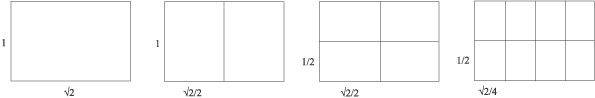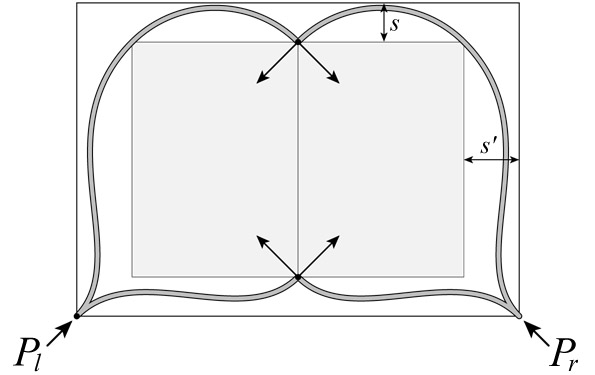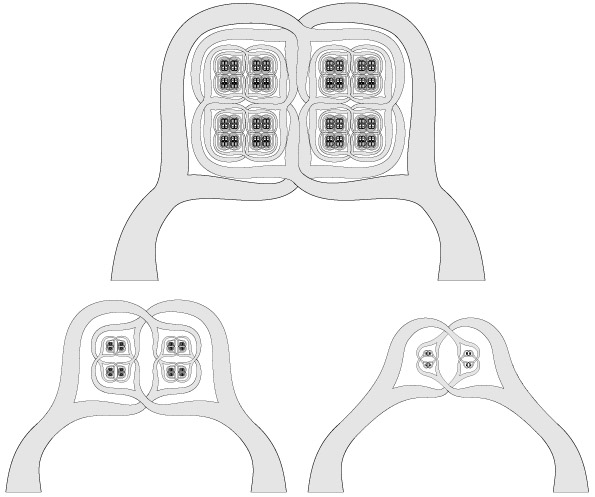Figures for "Rep-tiles with woven horns".

Cameron Browne
Nov 2004Figure 1. A pair of woven horns.Figure 2. Rep-2 rectangle progression.Figure 3. One iteration of recursive horn growth.Figure 4. Geometric construction of horn curves.Figure 5. Increasing iteration depth: d = 1, d = 2, d = 3, and d = 9.Figure 6. Spacing as a percentage of width: s = 10%, s = 20%, and s = 30%.